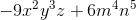# Algebra 1 : How to add polynomials

## Example Questions

← Previous 1 3 4 5

### Example Question #1 : How To Add Polynomials

Subtract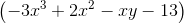from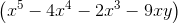.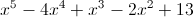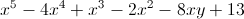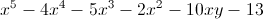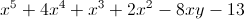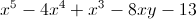Explanation:

Subtract the first expression from the second to get the following: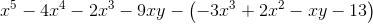This is equal to: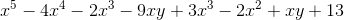Combine like terrms: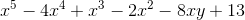### Example Question #1 : How To Add Polynomials

Simplify the following: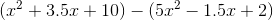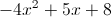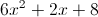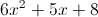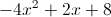Explanation: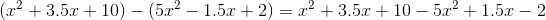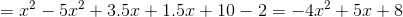### Example Question #1 : Simplifying Expressions

Simplify x(4 – x) – x(3 – x).

0

x

3x

1

x2

x

Explanation:

You must multiply out the first set of parenthesis (distribute) and you get 4x – x2. Then multiply out the second set and you get –3x + x2. Combine like terms and you get x.

x(4 – x) – x(3 – x)

4x – x2 – x(3 – x)

4x – x2 – (3x – x2)

4x – x2 – 3x + x2 = x

### Example Question #2 : Simplifying Polynomials

Simplify the following expression.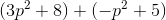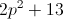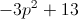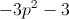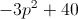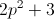Explanation:This is not a FOIL problem, as we are adding rather than multiplying the terms in parenteses.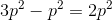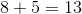Combining these terms into an expression gives us our answer.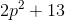### Example Question #4 : How To Add Polynomials

Simplify the expression.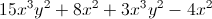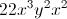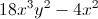None of the other answers are correct.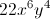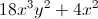Explanation:

When simplifying polynomials, only combine the variables with like terms.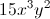can be added to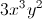, giving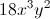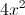can be subtracted from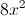to give.

Combine both of the terms into one expression to find the answer:### Example Question #5 : How To Add Polynomials

Simplify the following expression.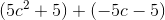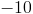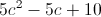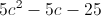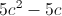Explanation:This is not a FOIL problem, as we are adding rather than multiplying the terms in parentheses.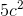and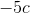have no like terms and cannot be combined with anything.

5 and -5 can be combined however: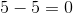This leaves us with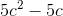.

### Example Question #6 : How To Add Polynomials

Find the LCM of the following polynomials: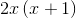,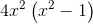,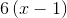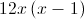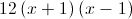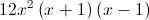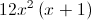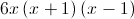Explanation:

LCM of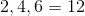LCM of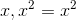and since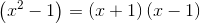The LCM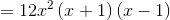### Example Question #7 : How To Add Polynomials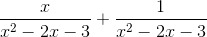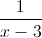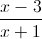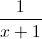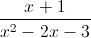Explanation:

First factor the denominators which gives us the following: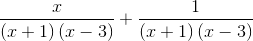The two rational fractions have a common denominator hence they are like "like fractions".  Hence we get: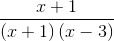Simplifying gives us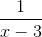### Example Question #8 : How To Add Polynomials

Simplify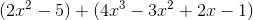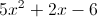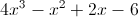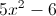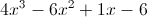Explanation:To simplify you combind like terms: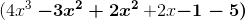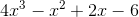### Example Question #2 : How To Add Polynomials

Combine: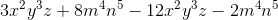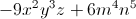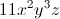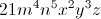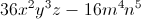When combining polynomials, only combine like terms. With the like terms, combine the coefficients. Your answer is